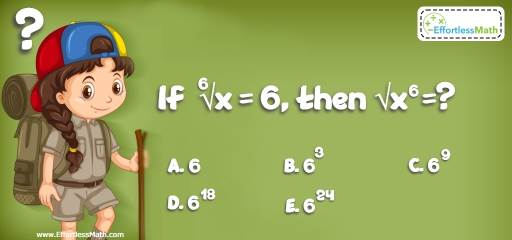# Number Properties Puzzle – Challenge 22

Check out this great math puzzle and enjoy a brain-bending challenge. The solution is also provided.## Challenge:

If $$\sqrt{x} = 6$$, then $$\sqrt{x^6}=$$?

A- $$6$$

B- $$6^3$$

C- $$6^{9}$$

D- $$6^{18}$$

E- $$6^{24}$$

### The Absolute Best Book to challenge your Smart Student!

$$\sqrt{x} = 6 → (\sqrt{x})^6 = 6^6 → X = 6^6$$
$$\sqrt{x^6} = \sqrt{(x^6)^6} = \sqrt{x^{36}} = x^{18}$$

### What people say about "Number Properties Puzzle – Challenge 22 - Effortless Math: We Help Students Learn to LOVE Mathematics"?

No one replied yet.

X
30% OFF

Limited time only!

Save Over 30%

SAVE $5 It was$16.99 now it is \$11.99